Nodal and Mesh Analysis
A sytematic approach to solving circuit problems

Overview of Nodal Analysis

Nodal Analysis is a step-by-step approach to solving circuits.
It is based on Kirchoff's Current Law.

With Nodal Analysis the node voltages of the circuit are the unknowns in the equations.
By solving for all the node voltages in a circuit, everything else can be found, from
currents to power calcuations. Knowing all the node voltages allows you to find anything else.

A set of independent equations are generated by applying KCL at each unknown node.
When a KCL equation is written at a node, the unknowns in the equation are node voltages.

All node voltages are expressed relative to a ground node, therefore one node is assigned
to be ground or zero volts.

In summary, Nodal Analysis allows one to quickly generate
as many independent equations as there are unknowns.

Once the equations have been generated, only algebra skills
(or linear algebra since these will be linear equations) are needed to finish the problem.

### Voltage is defined as existing between two nodes.

Even when we refer to the voltage at a specific node,
that voltage is always expressed relative to some other
node, typically our ground node. Ground is always zero
volts.

When expressing current using node voltages, we first find
the voltage difference between the two nodes.

voltage difference = V1 - V2
current = V/R = (voltage difference)/resistance

In the example shown:
iA = (V1 - V2)/R
or
iB = (V2 - V1)/R
Note that iA = -iB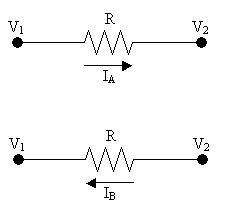Using this information, now we can generate a KCL equation at a node:

Consider this circuit segment. Generate a KCL equation at Node 1.

(V1 - V2)/R2 + (V1 - V3)/R3 + (V1 - V4)/R4 + (V1 - V5)/R5 = 0

Note that the above KCL equation has only node voltages as unknowns.

The equation is equivalent to:
` Σ Ileaving = 0 `
I2 + I3 + I4 + I5 = 0

#### When doing Nodal Analysis, always define currents as leaving the node.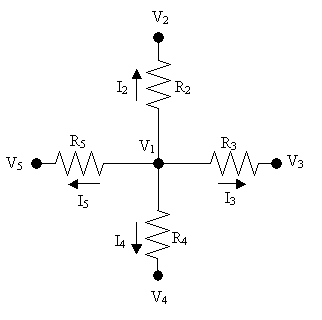#### Next let's look at how to generate independent equations using Nodal Analysis:

We will apply Nodal Analysis on this circuit:

We will generate enough independent equations for the
number of unknown node voltages.

This is done by writing a KCL equation at each unknown node.

### All parameters are node voltages when doing Nodal Analysis.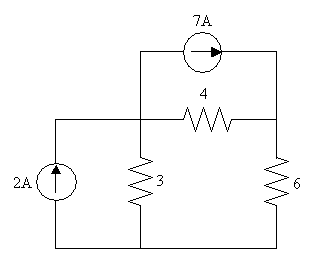Here is the same circuit labeled:

Step 1: Assign one node to be ground. You need a
reference node so that all other nodes are expressed relative
to the reference node. Note that V3 is defined as ground.
You can choose any node to be ground if a ground node does not already
exist.
Step 2: Count the number of unknown node voltages.
In this case we have three nodes but one is ground/zero.
Therefore we have two unknown nodes V1 and V2.
Step 3: Write a KCL equation at each unknown node.
Always let currents be leaving the node and express your equations
in terms of node voltages - NOT CURRENTS.

If you follow these steps you should generate as many equations
as you have unknowns:

KCL at V1:
-2 + (V1 - V3)/3 + (V1 - V2)/4 + 7 = 0
But V3 = 0, therefore

### -2 + V1/3 + (V1 - V2)/4 + 7 = 0

KCL at V2:
-7 + (V2 - V1)/4 + (V2 - V3)/6 = 0

### We now have two equations and two unknowns. All terms are in Amps. To find V1 and V2 at this point requires only algebra skills.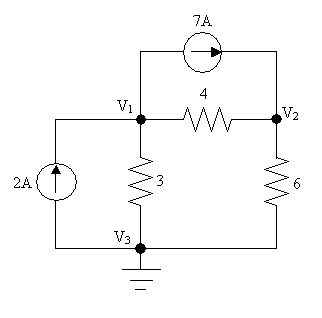## Study Problems

After clicking on the following link enter 5-1 for the problem and 1 for the step:
Study Problem 5-1
After clicking on the following link enter 5-2 for the problem and 1 for the step:
Study Problem 5-2

Top of Page

Applying Nodal Analysis on circuits containing voltage sources

When voltage sources exists in a problem where you wish to apply nodal analysis,
there are benefits as well as a new challenge.

The benefit is that the voltage sources give you information about the node voltages.

The new challenge is that you do not know the current through the voltage sources,
so if you apply KCL at a node which is connected to a voltage source, you must use
a supernode. This section shows you what a supernode is and how and why you use it.

#### Let's look at a circuit which contains a voltage source:

Here is a circuit with the nodes labeled and ground shown:

Note there are real benefits to having voltage sources in the
problem. By inspection we can get the following information:

V2 - V4 = 18V
Since V4 = 0 (ground is always zero)
Then V2 = 18V

Also by inspection we see that V1 - V3 = 6V

We have four nodes, but we already know V4 and V2
Also we already have one equation. Currently we have two unknowns
and already one equation, so we only need one more equation.
We will apply KCL at one of our unknown nodes
Let's try doing KCL at V3:

-2A + (V3 - V2)/3 + Ithrough voltage source = 0
But we do not know Ithrough voltage source.

### Therefore we need to draw a supernode.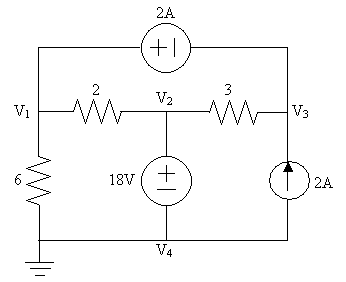Here we show the circuit with a supernode:

A supernode is a closed path enclosing part of the circuit. As with a node,

### `Σ ileaving supernode = ientering supernode`

In this particular circuit, all currents are shown as leaving the supernode. Therefore we can say:

### `Σ ileaving supernode = 0`

We can now apply KCL at the supernode to generate the following equation:
V1/6 + (V1 - V2)/2 + (V3 - V2)/3 + (-2A) = 0

We now have two equations and two unknowns, therefore now to solve for the node
voltages requires algebra skills only.

### In summary, we need to use a supernode when we can not find the current in a particular branch. By creating an area/supernode which encloses the branch, we avoid the branch.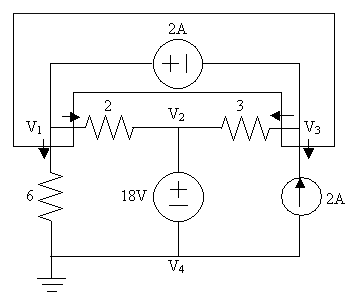### Use these steps to sytematically use Nodal Analysis when solving a circuit problem:

1. Find all nodes and label them
2. Choose one node to be ground (or zero). Try to pick the node with the
most connections to avoid a KCL equation with many unknowns.
This will make the math easier.
3. Write down everything you know by inspection. Voltage sources give you information about
node voltages.
4. If there are dependent sources in the problem, express their value in terms of node voltages.
5. Write a KCL equation for each unkonwn node. Always define current as leaving the node.
If there is a voltage source between two nodes then make a supernode around the voltage source.
6. After step 5 you should have N equations if you started with N unknowns. Using Algebra
or Linear Algebra you can now solve for all the node voltages.
7. If you know all the node voltages for the circuit, then anything else can be found (e.g. current
power, voltage across an element, etc.)

After clicking on the following link enter 5-3 for the problem and 1 for the step:
Study Problem 5-3

Top of Page

Overview of Mesh Analysis

Mesh Analysis is a step-by-step approach to solving circuits.
It is based on Kirchoff's Voltage Law.

With Mesh Analysis the mesh currents of the circuit are the unknowns in the equations.
By solving for all the mesh currents in a circuit, everything else can be found, from
voltages to power calcuations. Knowing all the mesh currents allows you to find anything else.

What is a mesh?
Think of a mesh as a window pane. If we have a planar circuit (one that is 2-dimensional)
then each "window pane" in the circuit is a mesh.

What is a mesh current?
A mesh current is the current flowing clockwise within a single mesh.

Here there are four meshes: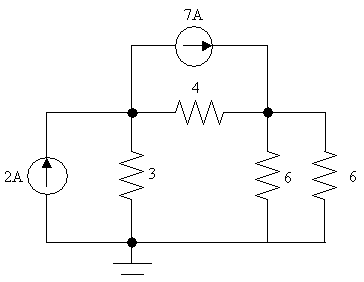Here there are five meshes: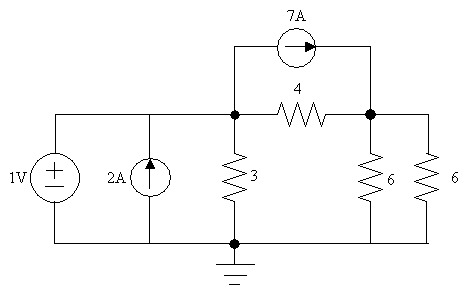This is not a planar circuit (wires cross other wires)
We can not apply Mesh Analysis to this circuit: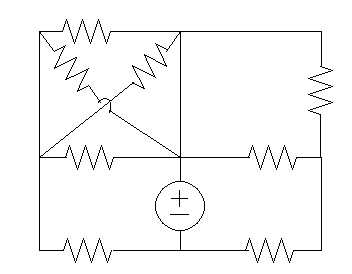A set of independent equations are generated by applying KVL in each mesh.
When a KVL equation is written in a mesh, the unknowns in the equation are mesh currents.

In summary, Mesh Analysis allows one to quickly generate
as many independent equations as there are unknowns.

Once the equations have been generated, only algebra skills
(or linear algebra since these will be linear equations) are needed to finish the problem.

### A mesh current is the current going clockwise within a mesh.

Here we have two meshes and therefore two mesh currents, I1 and I2.

When expressing voltage using mesh currents, we use Ohm's Law
which states V=IR if current is going from + to -.
In this case the current in the 6 Ohm going from + to - is (I1 - I2)

V = 6(I1 - I2)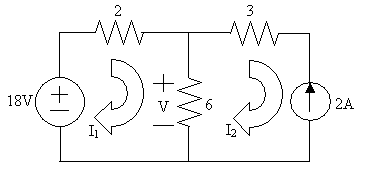Again we use Ohm's Law to calculate V.
In this case the current in the 6 Ohm going from + to - is (I2 - I1)

V = 6(I2 - I1)

This is exactly the opposite to the equation in the previous box.
This is because V is defined with an opposite polarity.
Both are correct!### Now let's do a problem using Mesh Analysis.

Our first step is to generate a KVL equation in each mesh:

Let's generate a KVL loop equation in mesh 1.

-18 + 2I1 + 6(I1 - I2) = 0

Note that the above KVL equation has only mesh currents as unknowns.

The equation is equivalent to:
` ΣVdrops - ΣVrises = 0 `
The equation reduces to: 8I1 - 6I2 = 18

Let's generate a KVL loop equation in mesh 2.

3I2 + 2 + 6(I2 - I1) = 0

As before the equation is equivalent to:
` ΣVdrops - ΣVrises = 0 `
The equation reduces to: 9I2 - 6I1 = -2

We now have two equations and two unkonwns.
Solving for I1 and I2 requires algebra.

#### When doing Mesh Analysis, always define mesh currents going clockwise in the mesh.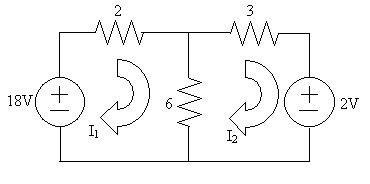### Next let's look at how to generate independent equations using the short-cut method:

We will apply Mesh Analysis on this circuit using the Short Cut Method:

We will generate enough independent equations for the
number of unknown mesh currents.

This is done by writing a KVL loop equation in each mesh
where the mesh current is unknown.

### All parameters are mesh currents when doing Mesh Analysis.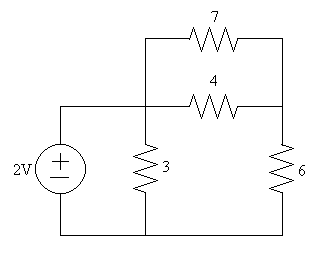### Here is the same circuit with all mesh currents labeled:

Step 1: Label all mesh currents and show mesh currents
going clockwise.
Step 2: Count the number of unknown mesh currents.
In this case we have three unknown mesh currents, I1, I2, & I3.
Step 3: Write a KVL equation in each mesh.
Express your equations in terms of mesh currents - NOT VOLTAGES.

The Short Cut Method is used to quickly/easily generate a
KVL loop equation for a mesh.

### To use the Short Cut Method to generate a KVL equation in a mesh, do the following:

1. Add up all the resistors in the mesh.
2. The coefficient for the mesh current will be the sum of all the resistors.
3. The coefficient for any other mesh current adjacent to the mesh will be
the negative of the resistance shared between the two meshes.
4. All terms generated in the first two steps will be added together and
equal to the sum of the voltages 'pushing' current in the clockwise direction.

### This is mush easier to show than to explain in English, so if this makes no sense see the solution below:

KVL in Mesh 1:
Adding up all the resistors in mesh 1 we get 3
So the coefficient for I1 is 3. Mesh 2 is adjacent to mesh 1, so the coefficient for I2 is -3.
The sum of the voltage sources pushing current in the clockwise direction is 2V.
Therefore the KVL equation is:

### 3I1 - 3I2 = 2

KVL in Mesh 2:
Adding up all the resistors in mesh 2 we get (3 + 4 + 6) = 13
So the coefficient for I2 is 13. Mesh 1 is adjacent to mesh 2, so the coefficient for I1 is -3.
Mesh 3 is adjacent to mesh 2, so the coefficient for I3 is -4.
The sum of the voltage sources pushing current in the clockwise direction is 0.
(There are no voltage sources in mesh 2.)
Therefore the KVL equation is:

### -3I1 + 13I2 - 4I3 = 0

KVL in Mesh 3:
Adding up all the resistors in mesh 3 we get (4 + 7) = 11
So the coefficient for I3 is 11. Mesh 2 is adjacent to mesh 3, so the coefficient for I2 is -4.
The sum of the voltage sources pushing current in the clockwise direction is 0.
(There are no voltage sources in mesh 3.)
Therefore the KVL equation is:

### -4I2 + 11I3 = 0

You should now solve for I1, I2, & I3
what you got in the previous section.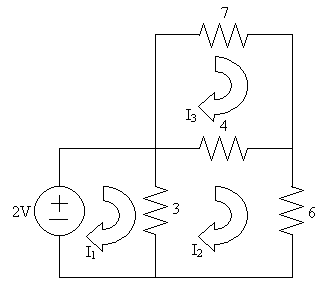Top of Page

Applying Mesh Analysis on circuits containing current sources

When current sources exists in a problem where you wish to apply mesh analysis,
there is a benefit as well as a new challenge.

The benefit is that the current sources give you information about the mesh currents.

The new challenge is that you do not know the voltages across the current sources,
so it is NOT possible to apply KVL in a mesh which contains a current source.
This section shows you how to use mesh analysis when current sources are present.

#### Let's look at a circuit which contains a current source:

We will apply mesh analysis to this circuit:

When a current source is present, it will give you information

When applying KVL to the circuit, you can temporarily remove the
current sources to 'see' which meshes don't have current sources.
It is in these meshes where you will write a KVL equation.

Note that sometimes there will not be enough meshes to generate the
required number of independent equations. In this case you will
have to resort to using a loop instead of just a mesh.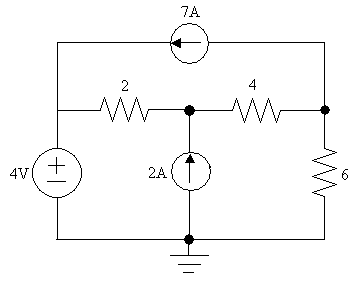### Here is the circuit labeled:

Step 1:
Looking at the current sources what information by inspection
can be acquired about the mesh currents?
I3 = -7A
I2 - I1 = 2A

Step 2:
Count the number of unknowns and the number of equations to determine
how many additional equations are needed.
We have two equations and three mesh currents. Therefore we need one
more equation.

Step 3:
Determine in which meshes it is possible to write a KVL in equation.
This can be determined by temporarily removing current sources to see
where closed paths exist. This is shown in the box below.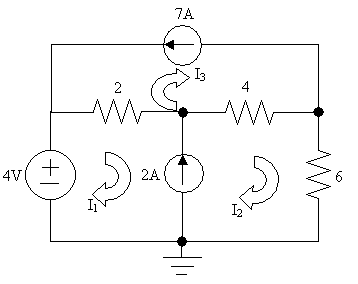Step 4:
Looking at the circuit on the right, there are no meshes
where KVL can be done. All the meshes have an open-circuit in them.

Therefore we have to resort to doing KVL in a loop to generate the
the independent equation that we need. There is only one loop here.

-4 + 2(I1-I3) + 4(I2-I3) + 6I2 = 0

We now have three equations and three unknowns.
Therefore all mesh currents can be found.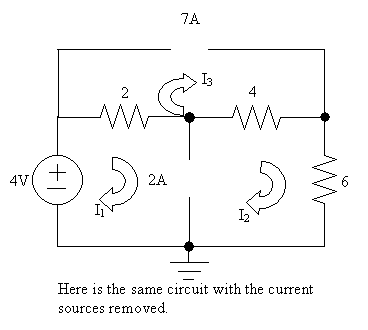### Use these steps to sytematically apply Mesh Analysis when solving a circuit problem:

1. Find all meshes and label them. Show mesh currents going clockwise.
2. Write down everything you know by inspection. Current sources give you information about
mesh currents. This means that you will not have to generate as many KVL equations.
3. If there are dependent sources in the problem, express their values in terms of mesh currents.
(There may be enough information to express the dependent source as a constant, which is even better.)
4. Write a KVL equation in each mesh where the mesh current is unknown.
You may have to temporarily remove current sources to visualize where the loops are.
5. After step 4 you should have N equations if you started with N unknowns. Using Algebra
or Linear Algebra you can now solve for all the mesh currents.
6. If you know all the mesh currents for the circuit, then anything else can be found (e.g. voltages,
power, current through an element, etc.)

After clicking on the following link enter 5-4 for the problem and 1 for the step:
Study Problem 5-4

Top of Page

How to decide which method to use

You now have two analysis techniques which allow you to generate
N independent equations for N unknowns.

When should you use Nodal Analysis and when should you use Mesh Analysis?

A good rule of thumb is if there are more unknown node voltages then there are
unknown mesh currents, then you should use Mesh. In other words, choose the method
with fewer unknowns.

An easy way to assess the circuit is to count the number of meshes and count
the number of nodes. Since one node is ground you can subtract one from the number
of nodes.

Then consider how many voltage sources there are because they help
generate equations for Nodal Analysis.
Similarly, consider how many current sources there are because they help
generate equations for Mesh Analysis.

After clicking on the following link enter 5-5 for the problem and 1 for the step:
Study Problem 5-5

Top of Page
Back To Index